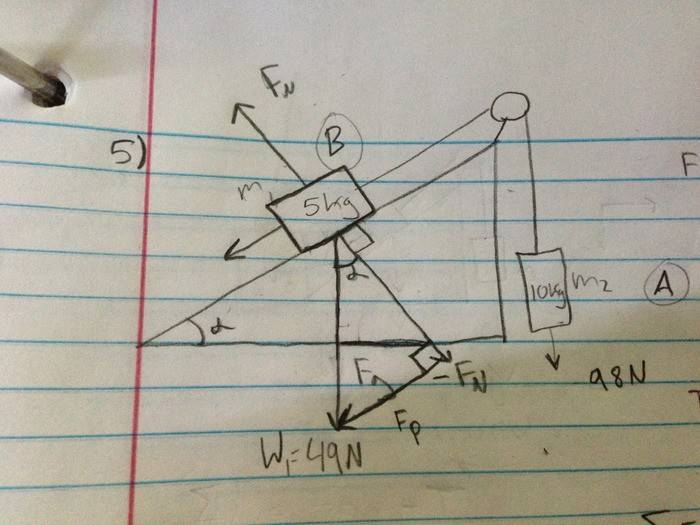# Pulley and ramp problem

## Homework Statement

A mass $m{1}$ = 5kg is on a frictionless ramp and connected to a cable that passes over a pulley which is then attached to a second hanging mass, $m{2}$ = 10kg. How long will it take the boxes to move 3m if they are released from rest?

$F{A}$= Forces acting on hanging object
$F{B}$= Forces acting on object on rampF=ma

## The Attempt at a Solution

I have solved for T using the force equation on object A and got T= -m1a + 98N

Then I substituted for T in the equation for object B and solved for acceleration, yielding:

a=(98N-49sin$\vartheta$)/15

My biggest problem is, the book says the answer is 1.13 seconds. How can I solve in terms of seconds without another variable provided? I can't think of a way to use elimination to solve for $\vartheta$.